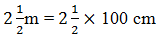Guru

# A ladder has rungs 25 cm apart. (see Fig. 5.7). The rungs decrease uniformly in length from 45 cm at the bottom to 25 cm at the top. If the top and the bottom rungs are Ncert solutions class 10 chapter 5-14 apart, what is the length of the wood required for the rungs? [Hint: Number of rungs = -250/25 ]. Q.3

• 0

The best way for solving the problem of question of arithmetic progressions of exercise 5.4 of class 10th how i solve this question in easy way A ladder has rungs 25 cm apart. (see Fig. 5.7). The rungs decrease uniformly in length from 45 cm at the bottom to 25 cm at the top. If the top and the bottom rungs are Ncert solutions class 10 chapter 5-14 apart, what is the length of the wood required for the rungs? [Hint: Number of rungs = -250/25 ].

Share

1. Given,

Distance between the rungs of the ladder is 25cm.

Distance between the top rung and bottom rung of the ladder is == 5/2 ×100cm

= 250cm

Therefore, total number of rungs = 250/25 + 1 = 11

As we can see from the figure, the ladder has rungs in decreasing order from top to bottom. Thus, we can conclude now, that the rungs are decreasing in an order of AP.

And the length of the wood required for the rungs will be equal to the sum of the terms of AP series formed.

So,

First term, a = 45

Last term, l = 25

Number of terms, n = 11

Now, as we know, sum of nth terms is equal to,

Sn= n/2(a+ l)

Sn= 11/2(45+25) = 11/2(70) = 385 cm

Hence, the length of the wood required for the rungs is 385cm.

• 0